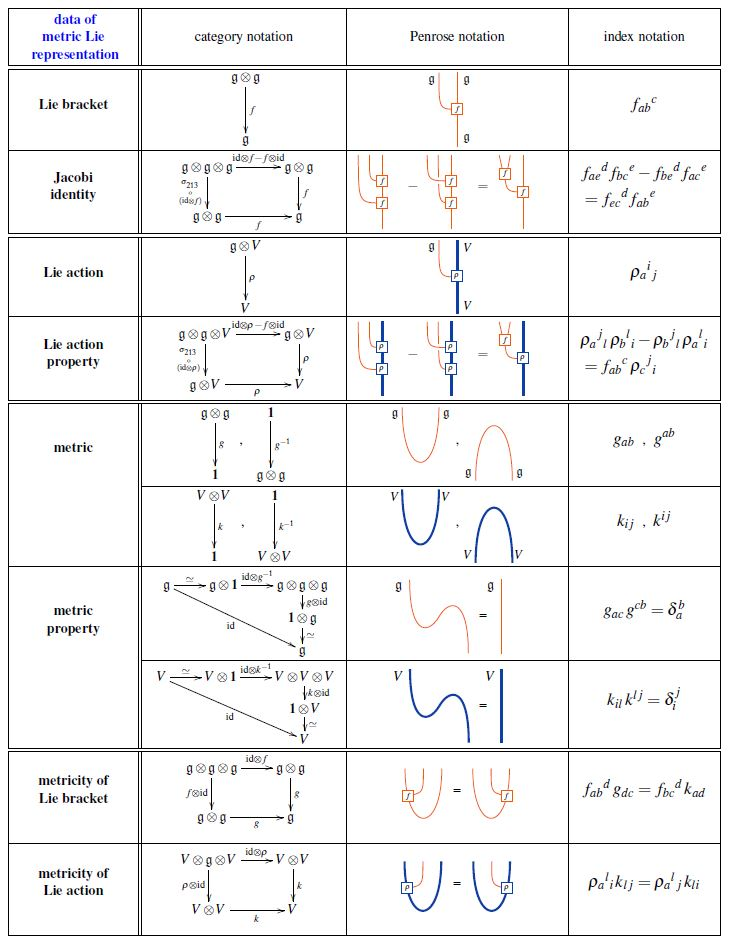# nLab metric Lie representation

Contents

### Context

#### Lie theory

Background

Smooth structure

Higher groupoids

Lie theory

∞-Lie groupoids

∞-Lie algebroids

Formal Lie groupoids

Cohomology

Homotopy

Related topics

Examples

$\infty$-Lie groupoids

$\infty$-Lie groups

$\infty$-Lie algebroids

$\infty$-Lie algebras

# Contents

## Idea

Given a Lie algebra $(\mathfrak{g}, [-,-])$ and a Lie algebra representation $\mathfrak{g} \otimes V \overset{\rho}{\to} V$, and given non-degenerate inner products, hence “metrics”, both on $\mathfrak{g}$ and on $V$, one may ask that all structure is compatible with these metrics. For the Lie algebra this means to have a metric Lie algebra and for the representation this means to have an orthogonal representation. Hence together this is an orthogonal representation of a metric Lie algebra, or metric Lie representation, for short.

## Definition

The following table shows the data in a metric Lie representation equivalently

1. in category theory-notation;

2. in index notation:graphics from Sati-Schreiber 19c

## Properties

### Structures induced from metric Lie representations

The following mathematical structures are induced from the data of metric Lie representations:

See there for more.

On the Faulkner construction (which became known as the M2-brane 3-algebra constructed from a metric Lie representation):